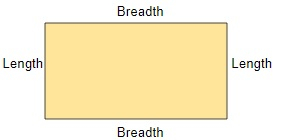# Maximum area of rectangle possible with given perimeter in C++

ProgrammingC++Server Side Programming

Given a perimeter of a rectangle, the task is to find the maximum area of the rectangle with that given perimeter.

A rectangle is a type of parallelogram whose opposite sides are equal and parallel.The perimeter of a rectangle is the sum of all sides of a rectangle; we can also say perimeter is the total distance of the outside of the rectangle.

The formula to find the perimeter of a rectangle is − Length + Breadth + Length + Breadth or 2(Length + Breadth)

Whereas the area of a rectangle is the size of the rectangular object. The formula for finding the area of a rectangle is − Length x Breadth.

So, to find the maximum area of the rectangle we have to get the length and breadth of the rectangle from its perimeter. We can find length and breadth by assuming Length as ceil(perimeter/4) and Breadth as floor(perimeter/4), this gives the maximum value of the length and breadth of a rectangle by its perimeter. Hence, the area of a rectangle will be, ceil(perimeter/4) * floor(perimeter/4).

Input − perimeter = 95

Output − The maximum area of rectangle possible with given perimeter 95 is − 552

Input − perimeter = 2.78

Output − The maximum area of rectangle possible with given perimeter 2.78 is − 0

## Approach used in the below program as  follows

• Take the perimeter of a rectangle as an input.

• Set result = ceil(perimeter/4) * floor(perimeter/4)

• Return and print the result.

## Example

Live Demo

#include <bits/stdc++.h>
using namespace std;
//calculate maximum area of rectangle possible with given perimeter
int Maximum(float perimeter){
int len = (int)ceil(perimeter / 4);
int bre = (int)floor(perimeter / 4);
// return area
return len* bre;
}
int main(){
float perimeter = 27;
cout<<"maximum area of rectangle possible with given perimeter "<<perimeter<<" is: "<<Maximum(perimeter);
return 0;
}

## Output

If we run the above code we will get the following output −

maximum area of rectangle possible with given perimeter 27 is: 42